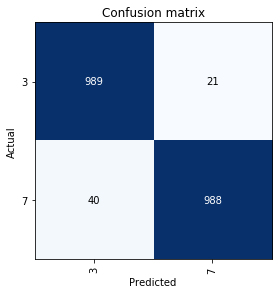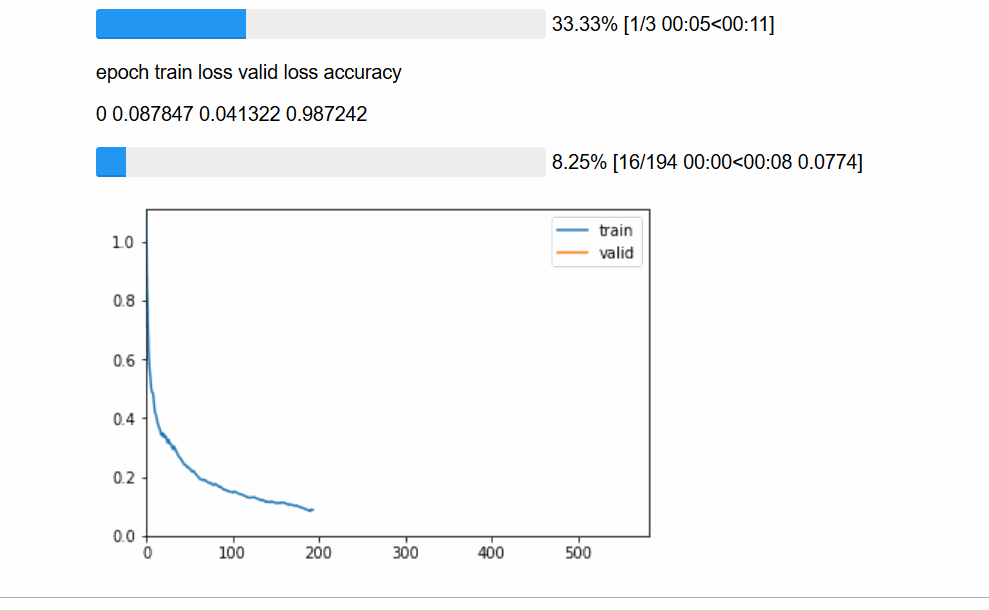Extensions to Learner that easily implement Callback

`train` provides a number of extension methods that are added to `Learner` (see below for a list and details), along with three simple callbacks:

## `Learner` extension methods¶

These methods are automatically added to all `Learner` objects created after importing this module. They provide convenient access to a number of callbacks, without requiring them to be manually created.

#### `fit_one_cycle`[source][test]

`fit_one_cycle`(`learn`:`Learner`, `cyc_len`:`int`, `max_lr`:`Union`[`float`, `Collection`[`float`], `slice`]=`slice(None, 0.003, None)`, `moms`:`Point`=`(0.95, 0.85)`, `div_factor`:`float`=`25.0`, `pct_start`:`float`=`0.3`, `final_div`:`float`=`None`, `wd`:`float`=`None`, `callbacks`:`Optional`[`Collection`[`Callback`]]=`None`, `tot_epochs`:`int`=`None`, `start_epoch`:`int`=`None`)

Tests found for `fit_one_cycle`:

• `pytest -sv tests/test_train.py::test_fit_one_cycle` [source]

Some other tests where `fit_one_cycle` is used:

• `pytest -sv tests/test_tabular_train.py::test_empty_cont` [source]
• `pytest -sv tests/test_text_train.py::test_qrnn_works_if_split_fn_provided` [source]
• `pytest -sv tests/test_text_train.py::test_qrnn_works_with_no_split` [source]

To run tests please refer to this guide.

Fit a model following the 1cycle policy.

#### `one_cycle_scheduler`[source][test]

`one_cycle_scheduler`(`lr_max`:`float`, **`kwargs`:`Any`) → `OneCycleScheduler`

No tests found for `one_cycle_scheduler`. To contribute a test please refer to this guide and this discussion.

Instantiate a `OneCycleScheduler` with `lr_max`.

#### `lr_find`[source][test]

`lr_find`(`learn`:`Learner`, `start_lr`:`Floats`=`1e-07`, `end_lr`:`Floats`=`10`, `num_it`:`int`=`100`, `stop_div`:`bool`=`True`, `wd`:`float`=`None`)

Tests found for `lr_find`:

• `pytest -sv tests/test_train.py::test_lr_find` [source]
• `pytest -sv tests/test_vision_train.py::test_lrfind` [source]

To run tests please refer to this guide.

Explore lr from `start_lr` to `end_lr` over `num_it` iterations in `learn`. If `stop_div`, stops when loss diverges.

See `LRFinder` for details.

#### `to_fp16`[source][test]

`to_fp16`(`learn`:`Learner`, `loss_scale`:`float`=`None`, `max_noskip`:`int`=`1000`, `dynamic`:`bool`=`True`, `clip`:`float`=`None`, `flat_master`:`bool`=`False`, `max_scale`:`float`=`16777216`, `loss_fp32`:`bool`=`True`) → `Learner`

No tests found for `to_fp16`. To contribute a test please refer to this guide and this discussion.

Put `learn` in FP16 precision mode.

See `MixedPrecision` for details.

#### `to_fp32`[source][test]

`to_fp32`(`learn`:`Learner`)

No tests found for `to_fp32`. To contribute a test please refer to this guide and this discussion.

Put `learn` back to FP32 precision mode.

#### `mixup`[source][test]

`mixup`(`learn`:`Learner`, `alpha`:`float`=`0.4`, `stack_x`:`bool`=`False`, `stack_y`:`bool`=`True`) → `Learner`

No tests found for `mixup`. To contribute a test please refer to this guide and this discussion.

Add mixup https://arxiv.org/abs/1710.09412 to `learn`.

See `MixUpCallback` for more details.

## `class``Interpretation`[source][test]

`Interpretation`(`learn`:`Learner`, `preds`:`Tensor`, `y_true`:`Tensor`, `losses`:`Tensor`, `ds_type`:`DatasetType`=`<DatasetType.Valid: 2>`)

No tests found for `Interpretation`. To contribute a test please refer to this guide and this discussion.

Interpretation base class, can be inherited for task specific Interpretation classes

#### `from_learner`[source][test]

`from_learner`(`learn`:`Learner`, `ds_type`:`DatasetType`=`<DatasetType.Valid: 2>`, `activ`:`Module`=`None`)

Tests found for `from_learner`:

Some other tests where `from_learner` is used:

• `pytest -sv tests/test_tabular_train.py::test_confusion_tabular` [source]
• `pytest -sv tests/test_vision_train.py::test_ClassificationInterpretation` [source]
• `pytest -sv tests/test_vision_train.py::test_interp` [source]

To run tests please refer to this guide.

Gets preds, y_true, losses to construct base class from a learner

#### `top_losses`[source][test]

`top_losses`(`k`:`int`=`None`, `largest`=`True`)

Tests found for `top_losses`:

Some other tests where `top_losses` is used:

• `pytest -sv tests/test_vision_train.py::test_interp` [source]
• `pytest -sv tests/test_vision_train.py::test_interp_shortcut` [source]

To run tests please refer to this guide.

`k` largest(/smallest) losses and indexes, defaulting to all losses (sorted by `largest`).

For example in `ClassificationInterpretation` is implemented using argmax on preds to set `self.pred_class` whereas an optional sigmoid is used for `MultilabelClassificationInterpretation`

## `class``ClassificationInterpretation`[source][test]

`ClassificationInterpretation`(`learn`:`Learner`, `preds`:`Tensor`, `y_true`:`Tensor`, `losses`:`Tensor`, `ds_type`:`DatasetType`=`<DatasetType.Valid: 2>`) :: `Interpretation`

Tests found for `ClassificationInterpretation`:

• `pytest -sv tests/test_vision_train.py::test_ClassificationInterpretation` [source]

Some other tests where `ClassificationInterpretation` is used:

• `pytest -sv tests/test_tabular_train.py::test_confusion_tabular` [source]
• `pytest -sv tests/test_vision_train.py::test_interp` [source]

To run tests please refer to this guide.

Interpretation methods for classification models.

```path = untar_data(URLs.MNIST_SAMPLE)
data = ImageDataBunch.from_folder(path)
learn = cnn_learner(data, models.resnet18)
learn.fit(1)
preds,y,losses = learn.get_preds(with_loss=True)
interp = ClassificationInterpretation(learn, preds, y, losses)
```

#### `top_losses`[source][test]

`top_losses`(`k`:`int`=`None`, `largest`=`True`)

Tests found for `top_losses`:

Some other tests where `top_losses` is used:

• `pytest -sv tests/test_vision_train.py::test_interp` [source]
• `pytest -sv tests/test_vision_train.py::test_interp_shortcut` [source]

To run tests please refer to this guide.

`k` largest(/smallest) losses and indexes, defaulting to all losses (sorted by `largest`).

Returns tuple of (losses,indices).

```torch.return_types.topk(
values=tensor([14.2152, 10.3850,  9.1650,  8.7286,  5.8163,  5.6689,  4.9013,  4.5471,
4.2432]),
indices=tensor([1059,  299,  960, 1831, 1775, 1467,  750, 1892,  634]))```

#### `plot_confusion_matrix`[source][test]

`plot_confusion_matrix`(`normalize`:`bool`=`False`, `title`:`str`=`'Confusion matrix'`, `cmap`:`Any`=`'Blues'`, `slice_size`:`int`=`1`, `norm_dec`:`int`=`2`, `plot_txt`:`bool`=`True`, `return_fig`:`bool`=`None`, **`kwargs`) → `Optional`[`Figure`]

No tests found for `plot_confusion_matrix`. To contribute a test please refer to this guide and this discussion.

Plot the confusion matrix, with `title` and using `cmap`.

If `normalize`, plots the percentages with `norm_dec` digits. `slice_size` can be used to avoid out of memory error if your set is too big. `kwargs` are passed to `plt.figure`.

```interp.plot_confusion_matrix()
```#### `confusion_matrix`[source][test]

`confusion_matrix`(`slice_size`:`int`=`1`)

Tests found for `confusion_matrix`:

• `pytest -sv tests/test_tabular_train.py::test_confusion_tabular` [source]

Some other tests where `confusion_matrix` is used:

• `pytest -sv tests/test_vision_train.py::test_ClassificationInterpretation` [source]

To run tests please refer to this guide.

Confusion matrix as an `np.ndarray`.

```interp.confusion_matrix()
```
```array([[989,  21],
[ 40, 988]])```

#### `most_confused`[source][test]

`most_confused`(`min_val`:`int`=`1`, `slice_size`:`int`=`1`) → `Collection`[`Tuple`[`str`, `str`, `int`]]

Tests found for `most_confused`:

Some other tests where `most_confused` is used:

• `pytest -sv tests/test_vision_train.py::test_ClassificationInterpretation` [source]

To run tests please refer to this guide.

Sorted descending list of largest non-diagonal entries of confusion matrix, presented as actual, predicted, number of occurrences.

## `class``MultiLabelClassificationInterpretation`[source][test]

`MultiLabelClassificationInterpretation`(`learn`:`Learner`, `preds`:`Tensor`, `y_true`:`Tensor`, `losses`:`Tensor`, `ds_type`:`DatasetType`=`<DatasetType.Valid: 2>`, `sigmoid`:`bool`=`True`, `thresh`:`float`=`0.3`) :: `Interpretation`

No tests found for `MultiLabelClassificationInterpretation`. To contribute a test please refer to this guide and this discussion.

Interpretation methods for classification models.

#### Working with large datasets¶

When working with large datasets, memory problems can arise when computing the confusion matrix. For example, an error can look like this:

``````RuntimeError: \$ Torch: not enough memory: you tried to allocate 64GB. Buy new RAM!

``````

In this case it is possible to force `ClassificationInterpretation` to compute the confusion matrix for data slices and then aggregate the result by specifying slice_size parameter.

```interp.confusion_matrix(slice_size=10)
```
```array([[989,  21],
[ 40, 988]])```
```interp.plot_confusion_matrix(slice_size=10)
``````interp.most_confused(slice_size=10)
```
`[('7', '3', 40), ('3', '7', 21)]`

We'll show examples below using our MNIST sample. As usual the `on_something` methods are directly called by the fastai library, no need to call them yourself.

```path = untar_data(URLs.MNIST_SAMPLE)
data = ImageDataBunch.from_folder(path)
```

### `class``ShowGraph`[source][test]

`ShowGraph`(`learn`) :: `LearnerCallback`

No tests found for `ShowGraph`. To contribute a test please refer to this guide and this discussion.

Update a graph of learner stats and metrics after each epoch.

```learn = cnn_learner(data, models.resnet18, metrics=accuracy, callback_fns=ShowGraph)
learn.fit(3)
```#### `on_epoch_end`[source][test]

`on_epoch_end`(`n_epochs`:`int`, `last_metrics`:`MetricsList`, **`kwargs`) → `bool`

No tests found for `on_epoch_end`. To contribute a test please refer to this guide and this discussion.

If we have `last_metrics` plot them in our pbar graph

## `class``GradientClipping`[source][test]

`GradientClipping`(`learn`:`Learner`, `clip`:`float`=`0.0`) :: `LearnerCallback`

No tests found for `GradientClipping`. To contribute a test please refer to this guide and this discussion.

```learn = cnn_learner(data, models.resnet18, metrics=accuracy,
learn.fit(1)
```
epoch train_loss valid_loss accuracy time
0 0.162001 0.100777 0.971050 00:07

#### `on_backward_end`[source][test]

`on_backward_end`(**`kwargs`)

No tests found for `on_backward_end`. To contribute a test please refer to this guide and this discussion.

Clip the gradient before the optimizer step.

## `class``BnFreeze`[source][test]

`BnFreeze`(`learn`) :: `LearnerCallback`

No tests found for `BnFreeze`. To contribute a test please refer to this guide and this discussion.

Freeze moving average statistics in all non-trainable batchnorm layers.

For batchnorm layers where `requires_grad==False`, you generally don't want to update their moving average statistics, in order to avoid the model's statistics getting out of sync with its pre-trained weights. You can add this callback to automate this freezing of statistics (internally, it calls `eval` on these layers).

```learn = cnn_learner(data, models.resnet18, metrics=accuracy, callback_fns=BnFreeze)
learn.fit(1)
```
epoch train_loss valid_loss accuracy time
0 0.163550 0.094137 0.971541 00:06

#### `on_epoch_begin`[source][test]

`on_epoch_begin`(**`kwargs`:`Any`)

No tests found for `on_epoch_begin`. To contribute a test please refer to this guide and this discussion.

Put bn layers in eval mode just after `model.train()`.

## `class``AccumulateScheduler`[source][test]

`AccumulateScheduler`(`learn`:`Learner`, `n_step`:`int`=`1`, `drop_last`:`bool`=`False`) :: `LearnerCallback`

No tests found for `AccumulateScheduler`. To contribute a test please refer to this guide and this discussion.

Does accumlated step every nth step by accumulating gradients

Let's force `batch_size=2` to mimic a scenario where we can't fit enough batch samples to our memory. We can then set `n_step` as desired to have an effective batch_size of `effective_batch_size=batch_size*n_step`.

It is also important to use loss func with `reduce='sum'` in order to calculate exact average accumulated gradients.

Another important note for users is that `batchnorm` is not yet adapted to accumulated gradients. So you should use this callback at your own risk until a hero fixes it :)

Here we demonstrate this callback with a model without `batchnorm` layers, alternatively you can use `nn.InstanceNorm` or `nn.GroupNorm`.

``````from torchvision.models import vgg11

data = ImageDataBunch.from_folder(path, bs=2)

learn = cnn_learner(data, resnet18, metrics=accuracy, loss_func=CrossEntropyFlat(reduction='sum'),
callback_fns=partial(AccumulateScheduler, n_step=16))
learn.fit(1)``````